# 1.2 Built-in Types of Data

A data type is a set of values and a set of operations defined on those values. Many data types are built into the Python language. In this section, we consider Python's built-in data types `int` (for integers), `float` (for floating-point numbers), `str` (for sequences of characters) and `bool` (for true-false values).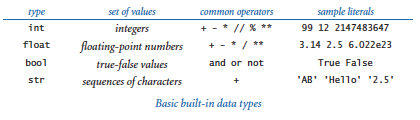## Definitions

To talk about data types, we need to introduce some terminology. To do so, we start with the following code fragment:

 ```a = 1234 b = 99 c = a + b ```

This code creates three objects, each of type `int`, using the literals `1234` and `99` and the expression `a + b`, and binds variables `a`, `b`, and `c` to those objects using assignment statements. The end result is that variable `c` is bound to an object of type `int` whose value is `1333`.

### Objects.

All data values in a Python program are represented by objects and relationships among objects. An object is an in-computer-memory representation of a value from a particular data type. Each object is characterized by its identity, type, and value.

• The identity uniquely identifies an object. You should think of it as the location in the computer's memory (or memory address) where the object is stored.
• The type of an object completely specifies its behavior — the set of values it might represent and the set of operations that can be performed on it.
• The value of an object is the data-type value that it represents.

Each object stores one value; for example, an object of type `int` can store the value `1234` or the value `99` or the value `1333`. Different objects may store the same value. For example, one object of type `str` might store the value `'hello'`, and another object of type `str` also might store the same value `'hello'`. We can apply to an object any of the operations defined by its type (and only those operations). For example, we can multiply two `int` objects but not two `str` objects.

### Object references.

An object reference is nothing more than a concrete representation of the object's identity (the memory address where the object is stored). Python programs use object references either to access the object's value or to manipulate the object references themselves.

### Literals.

A literal is a Python-code representation of a data-type value. It creates an object with the specified value.

### Operators.

An operator is a Python-code representation of a data-type operation. For example, Python uses `+` and `*` to represent addition and multiplication for integers and floating-point numbers; Python uses `and`, `or`, and `not` to represent boolean operations; and so forth.

### Identifiers.

An identifier is a Python-code representation of a name. Each identifier is a sequence of letters, digits, and underscores, the first of which is not a digit. The following keywords are reserved and you cannot use them as identifiers:

 ```False class finally is return None continue for lambda try True def from nonlocal while and del global not with as elif if or yield assert else import pass break except in raise ```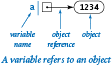### Variables.

A variable is a name for an object reference. We use variables to keep track of changing values as a computation unfolds. We use diagrams like the one at right to show the binding of a variable to an object.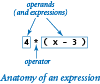### Expressions.

An expression is a combination of literals, variables, and operators that Python evaluates to produce an object. Each operand can be any expression, perhaps within parentheses. For example, we can compose expressions like `4 * (x - 3)` or `5 * x - 6` and Python will understand what we mean.

### Operator precedence.

An expression is shorthand for a sequence of operations. Python's precedence rules specify the order in which the operations should be applied. For arithmetic operations, multiplication and division are performed before addition and subtraction, so that `a - b * c` and `a - (b * c)` represent the same sequence of operations. When arithmetic operators have the same precedence, they are left associative, which means that `a - b - c` and `(a - b) - c` represent the same sequence of operations. You can use parentheses to override the rules, so you can write `a - (b - c)` if that is what you want. For full details see Appendix A: Operator Precedence in Python.

### Assignment statements.

An assignment statement is a directive to Python to bind the variable on the left side of the = operator to the object produced by evaluating the expression on the right side. For example, when we write `c = a + b`, we are expressing this action: "associate the variable `c` with the sum of the values associated with the variables `a` and `b`."

### Informal trace.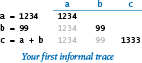An effective way to keep track of the values associated with variables is to use a table like the one at right, with one line giving the values after each statement has been executed. Such a table is called a trace.

### Object-level trace.

For a more complete understanding, we sometimes keep track of objects and references in traces. The object-level trace at right illustrates the full effect of our three assignment statements: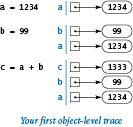• The statement `a = 1234` creates an `int` object whose value is `1234`; it then binds the variable `a` to this new int object.
• The statement `b = 99` creates an int object whose value is `99`; it then binds the variable `b` to this new `int` object.
• The statement `c = a + b` creates the int object whose value is `1333` as the sum of the value of the `int` object bound to `a` and the value of the `int` object bound to `b`; it then binds the variable `c` to the new `int` object.

## Strings

The `str` data type represents strings, for use in text processing.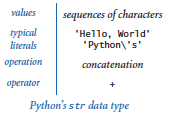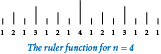The value of a `str` object is a sequence of characters. You can specify a `str` literal by enclosing a sequence of characters in matching single quotes. You can concatenate two strings using the operator `+`. As an example, ruler.py computes a table of values of the ruler function that describes the relative lengths of the marks on a ruler.

### Converting numbers to strings for output.

Python provides the built-in function `str()` to convert numbers to strings. Our most frequent use of the string concatenation operator is to chain together the results of a computation for output with `stdio.write()` and `stdio.writeln()`, often in conjunction with the `str()` function, as in this example:

 ```stdio.writeln(str(a) + ' + ' + str(b) + ' = ' + str(a+b)) ```

If `a` and `b` are `int` objects whose values are `1234` and `99`, respectively, then that statement writes the line of output `1234 + 99 = 1333`.

### Converting strings to numbers for input.

Python also provides built-in functions to convert strings (such as the ones we type as command-line arguments) to numeric objects. We use the Python built-in functions `int()` and `float()` for this purpose. If the user types `1234` as the first command-line argument, then the code `int(sys.argv)` evaluates to the `int` object whose value is `1234`.

## Integers

The `int` data type represents integers or natural numbers.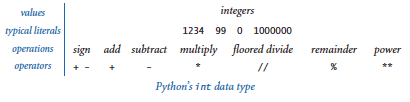Python includes operators for common arithmetic operations on integers, including `+` for addition, `-` for subtraction, `*` for multiplication, `//` for floored division, `%` for remainder, and `**` for exponentiation. All of these operators are defined just as in grade school (keeping in mind that the floored division operator results in an integer). Program intops.py illustrates basic operations for manipulating `int` objects.

### Division in Python 2.

In Python 3, the `/` operator has the same behavior as the floating-point division operator when both its operands are integers. In Python 2, the `/` operator has the same behavior as the floored division operator `//` when both its operands are integers. For example, `17 / 2` evaluates to `8.5` in Python 3 and to `8` in Python 2. For compatibility among Python versions, we do not use the `/` operator with two `int` operands in this booksite.

## Floating-Point Numbers

The `float` data type is for representing floating-point numbers, for use in scientific and commercial applications.Python includes operators for common arithmetic operations on floats, including + for addition, - for subtraction, * for multiplication, / for division, and ** for exponentiation. Program floatops.py illustrates the basic operations for manipulating `float` objects. Program quadratic.py shows the use of `float` objects in computing the two roots of a quadratic equation using the quadratic formula.

We use floating-point numbers to represent real numbers, but they are decidedly not the same as real numbers! There are infinitely many real numbers, but we can represent only a finite number of floating-point numbers in any digital computer. For example, `5.0/2.0` evaluates to `2.5` but `5.0/3.0` evaluates to `1.6666666666666667`. Typically, floating-point numbers have 15–17 decimal digits of precision.

Note the use of the `math.sqrt()` function in the quadratic.py program. The standard `math` module defines trigonometric functions, logarithm/exponential functions, and other common mathematical functions. To use the `math` module, place the statement `import math` near the beginning of the program and then call functions using syntax such as `math.sqrt(x)`.

## Booleans

The `bool` data type has just two values: `True` and `False`.The apparent simplicity is deceiving — booleans lie at the foundation of computer science. The most important operators defined for booleans are the logical ooperators: `and`, `or`, and `not`:

• `a` and `b` is `True` if both operands are `True`, and `False` if either is `False`.
• `a` or `b` is `False` if both operands are `False`, and `True` if either is `True`.
• `not a` is `True` if `a` is `False`, and `False` if `a` is `True`.

We can formally specify the definition of each operation using truth tables: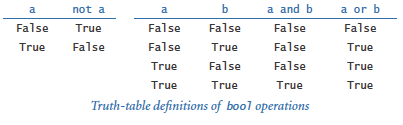## Comparisons

The comparison operators `==`, `!=`, `<`, `<=`, `>`, and `>=` are defined for both integers and floats, and evaluate to a boolean result.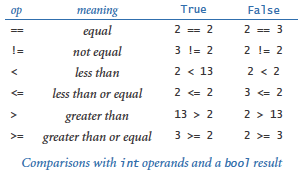The program leapyear.py shows the use of boolean expressions and comparison operations to compute whether a given year is a leap year. Section 1.3 of this booksite describes more common uses of the comparison operators.

## Functions and APIs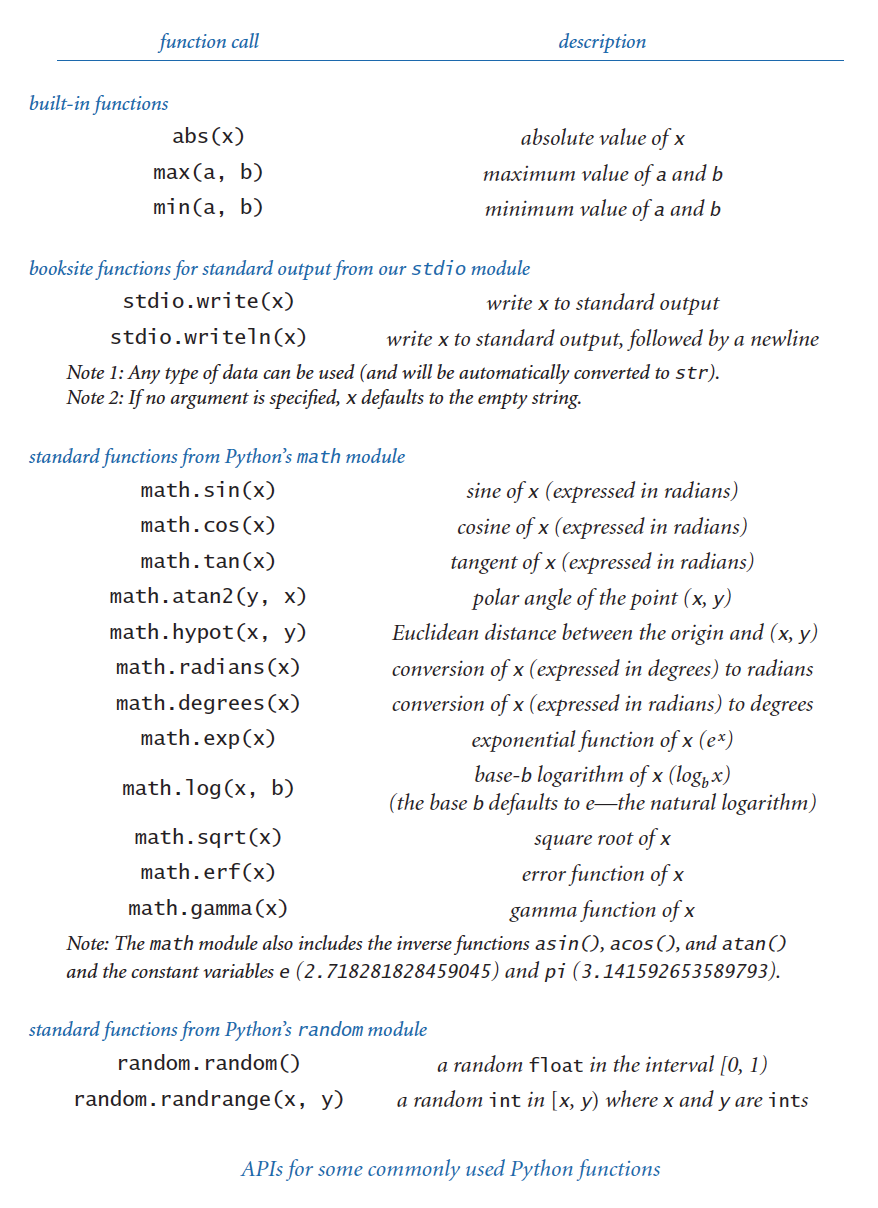As we have seen, many programming tasks involve using functions. We distinguish three kinds of functions: built-in functions (such as `int()`, `float()`, and `str()`) that you can use directly in any Python program, standard functions (such as `math.sqrt()`) that are defined in a Python standard module and are available in any program that imports the module, and booksite functions (such as `stdio.write()` and `stdio.writeln()`) that are defined in this booksite modules and available for you to use after you have made them available to Python and imported them. We describe some more useful functions in this section. In later chapters, you will learn not just how to use other functions, but how to define and use your own functions.

For convenience, we summarize the functions that you need to know how to use in tables like the one shown on the right. Such a table is known as an application programming interface (API). The table below shows some typical function calls.

## Type Conversion

We often find ourselves converting data from one type to another using one of the following approaches.

### Explicit type conversion.

Call functions such as `int()`, `float()`, `str()`, and `round()`.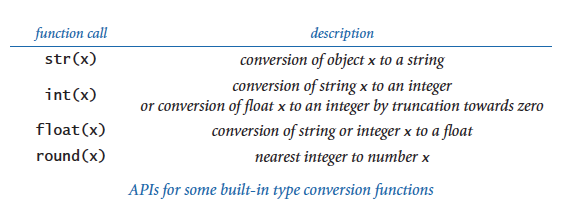### Implicit type conversion (from integer to float).

You can use an integer where a float is expected, because Python automatically converts integers to floats when appropriate. For example, `10/4.0` evaluates to `2.5` because `4.0` is a float and both operands need to be of the same type; thus, `10` is converted to a float and then the result of dividing two floats is a float. This kind of conversion is called automatic promotion or coercion.

## Interactive Python

If you issue the command `python` (that is, the word `python` stand-alone, with no following file name) in your terminal window, Python identifies itself and writes a `>>>` prompt. At that point you can type a Python statement and Python will execute it. Or, you can type a Python expression and Python will evaluate it and write the resulting value. Or, you can type `help()` to get access to Python's extensive interactive documentation. This is a convenient way to test new constructs and access documentation.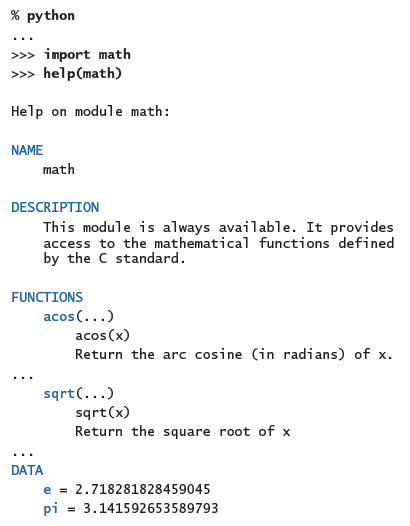#### Q & A: Strings

Q. How does Python store strings internally?

A. Strings are sequences of characters that are encoded with Unicode, a modern standard for encoding text. Unicode supports over 100,000 different characters, including more than 100 different languages plus mathematical and musical symbols.

Q. Which data type does Python provide for characters?

A. Python has no special data type for characters. A character is simply a string consisting of one element, such as `'A'`.

Q. Can I compare strings using comparison operators such as `==` and < or built-in functions such as `max()` and `min()`?

A. Yes. Informally, Python uses lexicographic order to compare two strings, like words in a book index or dictionary. For example, `'hello'` and `'hello'` are equal, `'hello'` and `'goodbye'` are unequal, and `'goodbye'` is less than `'hello'`.

Q. Can I use matching double quotes for string literals instead of single quotes?

A. Yes. For example, `'hello'` and `"hello"` are identical literals. Double quotes are useful to specify a string that contains single quotes, so that you don't need to escape them. For example, `'Python\'s'` and `"Python's"` are identical string literals. You can also use matching triple quotes for multiline strings. For example, the following creates a two-line string and assigns it to the variable `s`:

 ```s = """Python's "triple" quotes are useful to specify string literals that span multiple lines """ ```

In this booksite, we do not use double or triple quotes to delimit string literals.

 Strings in Python 2. Python 2 uses ASCII instead of Unicode to encode characters. ASCII is a legacy standard that supports 128 characters, including the English alphabet, numbers, and punctuation. Python 2 offers a separate data type unicode for strings composed of Unicode characters, but many Python 2 libraries do not support it.

#### Q & A: Integers

Q. How does Python store integers internally?

A. The simplest representation is for small positive integers, where the binary number system is used to represent each integer with a fixed amount of computer memory.

Q. What's the binary number system?

A. In the binary number system, we represent an integer as a sequence of bits. A bit is a single binary (base 2) digit — either 0 or 1 — and is the basis for representing information in computers. In this case the bits are coefficients of powers of 2. Specifically, the sequence of bits bnbn-1...b2b1b0 represents the integer

bn2n + bn-12n-1 + ... + b222 + b121 + b020

For example, 1100011 represents the integer

99 = 1 · 64 + 1 · 32 + 0 · 16 + 0 · 8 + 0 · 4 + 1 · 2 + 1 · 1.

The more familiar decimal number system is the same except that the digits are between zero and 9 and we use powers of 10. Converting a number to binary is an interesting computational problem that we will consider in the next section. For small integers, Python uses a fixed number of bits, typically dictated by a basic design parameter of your computer —usually 32 or 64. For example, the integer 99 might be represented with the 32 bits `00000000000000000000000001100011`.

Q. How about negative numbers?

A. Small negative numbers are handled with a convention known as two's complement, which we need not consider in detail. The definition of "small" depends on the underlying computer system. On older 32-bit machines, "small" typically covers the range -2147483648 (-231) to 2147483647 (231 - 1). On newer 64-bit machines, "small" typically covers the range -263 to 263 - 1, in which case "small" is not so small! If an integer is not "small," then Python automatically uses a more elaborate representation whose range is limited only by the amount of memory available on your computer system. Note that details of these internal representations are hidden from your programs, so you can use them in systems with different representations without having to change them.

Q. What does the expression `1/0` evaluate to in Python?

A. It raises a `ZeroDivisionError` at run time. Note: The easiest way to answer such questions is to use Python's interactive mode. Try it!

Q. How do the floored division operator `//` and remainder operator `%` work on negative operands?

A. Try them and see! `-47 // 5` evaluates to `-10` and `-47 % 5` evaluates to `3`. Generalizing, the floored division operator `//` yields the floored quotient; that is, the quotient is rounded toward minus infinity. The behavior of the remainder operator `%` is more complicated. In Python, if `a` and `b` are integers, then the expression `a % b` evaluates to an integer that has the same sign as `b`. This implies that `b * (a // b) + a % b == a` for any integers `a` and `b`. In some other languages (such as Java), the expression `a % b` evaluates to an integer that has the same sign as `a`.

Q. How does the exponentiation operator `**` work with negative operands?

A. Try it out and see for yourself. Note that the `**` operator has higher precedence than a unary plus/minus operator on its left but lower precedence than a unary plus/minus operator on its right. For example, `-3**4` evaluates to `-81` (and not `81`). Also, it can result in an object of a different type. For example, `10**-2` evaluates to the float `0.01` and `(-10)**(10**-2)` evaluates to a complex number in Python 3 (but raises a run-time error in Python 2).

Q. Why does `10^6` evaluate to `12` instead of `1000000`?

A. The `^` operator is not an exponentiation operator, which you must have been thinking. Instead, it is an operator that we do not use in this book. You want the literal `1000000`. You could use the expression `10**6`, but it is wasteful to use an expression (which requires evaluation at run time) when a literal would suffice.

 Integers in Python 2. Python 2 supports two separate types for integers — `int` (for small integers) and `long` (for larger integers). Python 2 automatically promotes from type `int` to `long` whenever necessary.

#### Q & A: Floating point numbers

Q. Why is the type for real numbers named `float`?

A. The decimal point can "float" across the digits that make up the real number. In contrast, with integers the (implicit) decimal point is fixed after the least significant digit.

Q. How does Python store floating-point numbers internally?

A. Generally, Python uses the representation that is natural for the underlying computer system. Most modern computer systems store floating-point numbers as defined by the IEEE 754 standard. That standard specifies that a floating-point number is stored using three fields: sign, mantissa, and exponent. If you are interested, see the the Wikipedia IEEE floating point page for more details. The IEEE 754 standard also specifies how special floating-point values — positive zero, negative zero, positive infinity, negative infinity, and `NaN` (not a number) — should be handled. For example, it specifies that `-0.0/3.0` should evaluate to `-0.0`, `1.0/0.0` should evaluate to positive infinity, and `0.0/0.0` should evaluate to `NaN`. You can use the (rather unusual) expressions `float('inf')` and `float('-inf')` for positive and negative infinity in some simple calculations, but Python does not conform to this part of the IEEE 754 standard. For example, in Python, `-0.0/3.0` correctly evaluates to `-0.0`, but both `1.0/0.0` and `0.0/0.0` raise a `ZeroDivisionError` at run time.

Q. Fifteen digits for floating-point numbers certainly seems enough to me. Do I really need to worry much about precision?

A. Yes, because you are used to mathematics based on real numbers with infinite precision, whereas the computer always deals with approximations. For example, in IEEE 754 floating point, the expression `(0.1 + 0.1 == 0.2)` evaluates to `True` but `(0.1 + 0.1 + 0.1 == 0.3)` evaluates to `False`! Pitfalls like this are not at all unusual in scientific computing. Novice programmers should avoid comparing two floating-point numbers for equality.

Q. It is annoying to see all those digits when writing a float. Is it possible to get `stdio.write()` and `stdio.writeln()` to write just two or three digits after the decimal point?

A. The booksite function `stdio.writef()` is one way to do the job — it is similar to the basic formatted writing function in the C programming language and many other modern languages, as discussed in Section 1.5. Until then, we will live with the extra digits (which is not all bad, since doing so helps us to get used to the different types of numbers).

Q. Can I apply the floored division operator `//` to two float operands?

A. Yes, it produces the floored division of its operands. That is, the result is the quotient in which digits after the decimal place are removed. We do not use the floored division operator on floats in this book.

Q. What does `round()` return if the fractional part of its argument is `0.5`?

A. In Python 3, it returns the nearest even integer, so `round(2.5)` is `2`, `round(3.5)` is `4`, and `round(-2.5)` is `-2`. But in Python 2, the `round()` function rounds away from zero (and returns a float), so `round(2.5)` is `3.0`, `round(3.5)` is `4.0`, and `round(-2.5)` is `-3.0`.

Q. Can I compare a `float` to an `int`?

A. Not without doing a type conversion, but remember that Python does the requisite type conversion automatically. For example, if `x` is the integer `3`, then the expression `(x < 3.1)` evaluates to `True` because Python promotes the integer `3` to generate the float `3.0` and then compares `3.0` with `3.1`.

Q. Are there functions in Python's `math` module for other trigonometric functions, such as arc sine, hyperbolic sine, and secant?

A. Yes, Python's `math` module includes inverse trigonometric functions and hyperbolic functions. However, there are no functions for secant, cosecant, and cotangent because you could use `math.sin()`, `math.cos()`, and `math.tan()` to compute them easily. Choosing which functions to include in an API is a tradeoff between the convenience of having every function that you need and the annoyance of having to find one of the few that you need in a long list. No choice will satisfy all users, and the Python designers have many users to satisfy. Note that there are plenty of redundancies even in the APIs that we have listed. For example, you could use `math.sin(x) / math.cos(x)` instead of `math.tan(x)`.

#### Q & A

Q. What happens if I access a variable that I haven't bound to an object?

A. Python will raise a `NameError` at run time.

Q. How can I determine the type of a variable?

A. That's a trick question. Unlike variables in many programming languages (such as Java), a Python variable does not have a type. Instead, it is the object to which a variable is bound that has a type. You can bind the same variable to objects of different types, as in this code fragment:
 ```x = 'Hello, World' x = 17 x = True ```

However, for clarity, it's usually a bad idea to do so.

Q. How can I determine the type, identity, and value of an object?

A. Python provides built-in functions for this purpose. The function `type()` returns the type of an object; the function `id()` returns the identity of an object; the function `repr()` returns an unambiguous string representation of an object.
 ```>>> import math >>> a = math.pi >>> id(a) 140424102622928 >>> type(a) >>> repr(a) '3.141592653589793' ```

You will rarely use these functions in ordinary programming, but you may find them useful when debugging.

Q. Is there a difference between `=` and `==` ?

A. Yes, they are quite different! The first specifies an assignment to a variable, and the second is a comparison operator that produces a boolean result. Your ability to understand this answer is a sure test of whether you understood the material in this section. Think about how you might explain the difference to a friend.

Q. Will `a < b < c` test whether the three numbers `a`, `b`, and `c` are in order?

A. Yes, Python supports arbitrary chaining of comparisons such as `a < b < c` that behave according to standard mathematical conventions. However, in many programming languages (such as Java) the expression `a < b < c` is illegal because the subexpression `a < b` evaluates to a boolean and that boolean is then compared with a number, which is meaningless. We do not use chained comparisons in this book; instead we prefer expressions such as `(a < b) and (b < c)`.

Q. Will `a = b = c = 17` set the three variables to 17?

A. Yes, even though Python assignment statements are not expressions, Python supports arbitrary chaining of assignment statements. We do not use chained assignments in the book because many Python programmers consider it poor style.

Q. Can I use the logical operators `and`, `or`, and `not` with operands that are not booleans?

A. Yes, but for clarity it's usually a bad idea to do so. In this context, Python considers `0`, `0.0`, and the empty string `''` to mean `False`, and any other integer, float, or string to mean `True`.

Q. Can I use arithmetic operators with boolean operands?

A. Yes, but again it's bad form to do so. When you use boolean operands with arithmetic operators, they are promoted to integers: `0` for `False` and `1` for `True`. For example, `(False - True - True) * True` evaluates to the `int` value `-2`.

Q. Can I name a variable `max`?

A. Yes, but if you do, then you won't be able to use the built-in function `max()`. The same holds for `min()`, `sum()`, `float()`, `eval()`, `open()`, `id()`, `type()`, `file()`, and other built-in functions.

#### Exercises

1. Suppose that `a` and `b` are integers. What does the following sequence of statements do? Draw an object-level trace of this computation.

 ```t = a b = t a = b ```

Solution: The sequence sets `a`, `b`, and `t` to the original value of `a`.

2. Compose a program that uses `math.sin()` and `math.cos()` to check that the value of cos2 θ + sin2 θ is approximately `1.0` for any θ entered as a command-line argument. Just write the value. Why are the values not always exactly `1.0`?

3. Solution (from Hassan Alam and Lee Jong Gil):

```import sys
import math

theta = float(sys.argv)

result = (val_of_sin**2) + (val_of_cos**2)
sys.stdout.write(str(result))
```
4. Suppose that `a` and `b` are booleans. Show that this expression evaluates to `True`:

 ```(not (a and b) and (a or b)) or ((a and b) or not (a or b)) ```
5. Suppose that `a` and `b` are integers. Simplify the following expression:

 ```(not (a < b) and not (a > b)) ```

Solution: `a == b`

6. What does each of these statements write? Explain each outcome.

 ```stdio.writeln(2 + 3) stdio.writeln(2.2 + 3.3) stdio.writeln('2' + '3') stdio.writeln('2.2' + '3.3') stdio.writeln(str(2) + str(3)) stdio.writeln(str(2.2) + str(3.3)) stdio.writeln(int('2') + int('3')) stdio.writeln(int('2' + '3')) stdio.writeln(float('2') + float('3')) stdio.writeln(float('2' + '3')) stdio.writeln(int(2.6 + 2.6)) stdio.writeln(int(2.6) + int(2.6)) ```
7. Explain how to use quadratic.py to find the square root of a number.

Solution: To find the square root of `c`, find the roots of `x2 + 0x - c`.

8. What does `stdio.writeln((1.0 + 2 + 3 + 4) / 4)` write?

9. Suppose that `a` is `3.14159`. What do each of these statements write? Explain each outcome.

 ```stdio.writeln(a) stdio.writeln(a + 1.0) stdio.writeln(8 // int(a)) stdio.writeln(8.0 / a) stdio.writeln(int(8.0 / a)) ```
10. Describe the effect of writing `sqrt` instead of `math.sqrt` in quadratic.py.

11. Does (math.sqrt(2) * math.sqrt(2) == 2) evaluate to True or False?

12. Compose a program that takes two positive integers as command-line arguments and writes `True` if either evenly divides the other.

13. Compose a program that takes three positive integers as command-line arguments and writes `False` if any one of them is greater than or equal to the sum of the other two and `True` otherwise. (Note: This computation tests whether the three numbers could be the lengths of the sides of some triangle.)

14. Give the value of `a` after the execution of each of the following sequences:

 ```a = 1 a = True a = 2 a = a + a a = not a a = a * a a = a + a a = not a a = a * a a = a + a a = not a a = a * a ```
15. A physics student gets unexpected results when using the code

 ```force = G * mass1 * mass2 / radius * radius ```

to compute values according to the formula F = Gm1m2 / r2. Explain the problem and correct the code.

Solution: The code divides by `r`, and then multiplies by `r`. Instead it should divide by `r` squared. Use parentheses:

 ```F = G * mass1 * mass2 / (r * r) ```

Or use the exponentiation operator:

 ```F = G * mass1 * mass2 / r ** 2 ```

Or, better still for the sake of clarity, use both parentheses and the exponentiation operator:

 ```F = (G * mass1 * mass2) / (r ** 2) ```
16. Suppose that `x` and `y` are two floats that represent the Cartesian coordinates of a point (x, y) in the plane. Give an expression that evaluates to the distance of the point from the origin.

Solution: `math.sqrt(x*x + y*y)`

17. Compose a program that takes two integers `a` and `b` from the command line and writes a random integer between `a` and `b`.

18. Compose a program that writes the sum of two random integers between 1 and 6 (such as you might get when rolling dice).
19. Solution: See sumoftwodice.py.

20. Compose a program that takes a float `t` from the command line and writes the value of sin(2t) + sin(3t).

21. Compose a program that takes three floats x0, v0, and t from the command line, evaluates x0 + v0t - Gt2 / 2, and writes the result. (Note: G is the constant 9.80665. This value is the displacement in meters after t seconds when an object is thrown straight up from initial position x0 at velocity v0 meters per second.)

22. Compose a program that takes two integers `m` and `d` from the command line and writes `True` if day d of month m is between March 20 and June 20, and False otherwise. (Interpret m with 1 for January, 2 for February, and so forth.)

Solution: See spring.py.

#### Creative Exercises

1. Continuously compounded interest. Compose a program that calculates and writes the amount of money you would have if you invested it at a given interest rate compounded continuously, taking the number of years t, the principal P, and the annual interest rate r as commmand-line arguments. The desired value is given by the formula pert.

2. Wind chill. Given the temperature t (in Fahrenheit) and the wind speed v (in miles per hour), the National Weather Service defines the effective temperature (the wind chill) to be:

 w = 35.74 + 0.6215 t + (0.4275 t - 35.75) v0.16

Compose a program that takes two floats `t` and `v` from the command-line and writes the wind chill. Note: the formula is not valid if t is larger than 50 in absolute value or if v is larger than 120 or less than 3 (you may assume that the values you get are in that range).

Solution: See windchill.py.

3.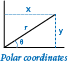4. Polar coordinates. Compose a program that converts from Cartesian to polar coordinates. Your program should accept two floats `x` and `y` from the command-line and write the polar coordinates r and θ. Use the Python function `math.atan2(y, x)`, which computes the arctangent value of y/x that is in the range from to `π`.

Solution: See polar.py.

5. Gaussian random numbers. One way to generate a random number taken from the Gaussian distribution is to use the Box-Muller formula:

 Z = sin(2 π v) (-2 ln u)1/2

where u and v are real numbers between 0 and 1 generated by the `random.random()` function. Compose a program that writes a standard Gaussian random variable.

6. Order check. Compose a program that takes three floats `x`, `y`, and `z` as command-line arguments and writes `True` if the values are strictly ascending or descending (`x < y < z` or `x > y > z`), and `False` otherwise.

7. Day of the week. Compose a program that accepts a date as input and writes the day of the week on which that date falls. Your program should accept three command-line arguments: `m` (month), `d` (day), and `y` (year). For `m` use 1 for January, 2 for February, and so forth. For output write 0 for Sunday, 1 for Monday, 2 for Tuesday, and so forth. Use the following formulas for the Gregorian calendar:

 y0 = y - (14 - m) / 12 x = y0 + y0/4 - y0/100 + y0/400 m0 = m + 12 * ((14 - m) / 12) - 2 d0 = (d + x + (31*m0)/ 12) mod 7

For example, on what day of the week was August 2, 1953?

 y = 1953 - 0 = 1953 x = 1953 + 1953/4 - 1953/100 + 1953/400 = 2426 m = 8 + 12*0 - 2 = 6 d = (2 + 2426 + (31*6) / 12) mod 7 = 2443 mod 7 = 0 (Sunday)

Solution: See day.py.

8. Uniform random numbers. Compose a program that writes five uniform random floats between 0 and 1, their average value, and their minimum and maximum value. Use the built-in `max()` and `min()` functions.

Solution: See stats1.py.

9. Mercator projection. The Mercator projection is a conformal (angle preserving) projection that maps latitude φ and longitude λ to rectangular coordinates (x, y). It is widely used — for example, in nautical charts and in the maps that you print from the web. The project is defined by the equations:

10.  x = λ - λ0 y = 1/2 * ln((1 + sin(φ)) / (1 - sin(φ)))

where λ0 is the longitude of the point in the center of the map. Compose a program that accepts λ0 and the latitude and longitude of a point from the command line and writes its projection.

11. Color conversion. Several different formats are used to represent color. For example, the primary format for LCD displays, digital cameras, and web pages, known as the RGB format, specifies the level of red (R), green (G), and blue (B) on an integer scale from 0 to 255. The primary format for publishing books and magazines, known as the CMYK format, specifies the level of cyan (C), magenta (M), yellow (Y), and black (K) on a real scale from 0.0 to 1.0. Compose a program that converts RGB to CMYK. Accept three integers —r, g, and b —from the command line and write the equivalent CMYK values. If the RGB values are all 0, then the CMY values are all 0 and the K value is 1; otherwise, use these formulas:

 w = max(r/255, g/255, b/255) c = (w - r/255) / w m = (w - g/255) / w y = (w - b/255) / w k = 1 - w

Here's an example run:

```\$ python rgbtocmyk.py 75 0 130       # indigo
cyan    = 0.4230769230769229
magenta = 1.0
yellow  = 0.0
black   = 0.4901960784313726
```
12.13. Great circle. Compose a program that takes four float command-line arguments `x1`, `y1`, `x2`, and `y2` (the latitude and longitude, in degrees, of two points on the earth) and writes the great-circle distance between them. The great-circle distance `d` (in nautical miles) is given by the formula derived from the law of cosines:

 d = 60 * arccos(sin(x1) * sin(x2) + cos(x1) * cos(x2) * cos(y1 - y2))

Note that this equation uses degrees, whereas Python's trigonometric functions use radians. Use `math.radians()` and `math.degrees()` to convert between the two. Use your program to compute the great-circle distance between Paris (48.87° N, -2.33° W) and San Francisco (37.8° N, 122.4° W).

Note: the shape of the earth is more like a flattened spheroid than a sphere, so the formula above is only an approximation (up to around 0.5% error). Also, this formula is unreliable for small distances because the inverse cosine function is ill-conditioned.

Here is the Haversine formula:

 a = sin2((L2-L1)/2) + cos(L1) * cos(L2) * sin2((G2-G1)/2) c = 2 * arcsin(min(1, sqrt(a))) # distance in radians distance = 60 * c # nautical miles

The Haversine formula is accurate for most distances, but it suffers from rounding errors when the points are (nearly) antipodal. The following formula is accurate for all distances.

 delta = G1 - G2 p1 = cos(L2) * sin(delta) p2 = cos(L1) * sin(L2) - sin(L1) * cos(L2) * cos(delta) p3 = sin(L1) * sin(L2) + cos(L1) * cos(L2) * cos(delta) distance = 60 * atan2(sqrt(p1*p1 + p2*p2), p3)

This Kahan reference provides more details.

Solution: See greatcircle.py.

14. Three-sort. Compose a program that accepts three integers from the command line and writes them in ascending order. Use the built-in `min()` and `max()` functions.

Solution: See threesort.py.

15.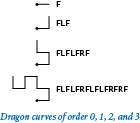16. Dragon curve. Compose a program to write the instructions for drawing the dragon curves of order 0 through 5. The instructions are strings of the characters `F`, `L`, and `R`, where `F` means "draw line while moving 1 unit forward", `L` means "turn left", and `R` means turn right. A dragon curve of order `n` is formed when you fold a strip of paper in half `n` times, then unfold to right angles. The key to solving this problem is to note that a curve of order n is a curve of order n-1 followed by an L followed by a curve of order n-1 traversed in reverse order, and then to figure out a similar description of the reverse curve.

Solution: See dragon1.py.